# Number

The concept of number is one of the most elementary, or fundamental notions of mathematics. Such elementary concepts cannot be defined in terms of other concepts (trivially, if an elementary concept could be defined in terms of other concepts, then it would not in fact be fundamental). Rather, a fundamental concept such as number can only be explained by demonstration. Such an approach relies for its efficacy on the intuitive properties of the human mind and its ability to abstract and generalize.

There are philosophical problems bound up with the concept of number. First, there is the ontological problem of the various types of numbers — do they exist, or are they "mental concepts". Then there is the epistemological problem which is concerned with how we know anything about numbers.

In mathematics, a number is formally a member of a given set (possibly an ordered set). It conveys the ideas of :

However, due to the expressiveness of positional number systems, the usefulness of geometric objects, and the advances in different scientific fields, it can convey more properties.

A word written only with digits is called a numeral, and may represent a number. Numerals are often used for labeling (like telephone numbers), for ordering (like serial numbers), and for encoding (like ISBNs).

The writing of a number depends on the numeral system in use. For instance, the number 12 is written "1100" in base 2, "C" in base 16, and "XII" as a roman numeral. We can geometrically represent a number with unitless vectors in a cartesian system or by drawing simple shapes (e.g., squares and circles). There are other means to express a number.

Abstract algebra studies abstract number systems such as groups, rings and fields.

##  Number sets

This section presents different number sets, but this list is not exhaustive.

1. The natural numbers ($\mathbb{N}$) are used to count things (e.g., there are 52 weeks in a Julian year). This set contains many remarkable subsets : prime numbers, Fibonacci numbers, perfect numbers, catalan numbers, etc.
2. The integers (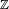$\mathbb{Z}$) also include negative numbers, that can be used to represent debits and credits, etc. (e.g., a company owes 60 millions US dollars to a bank). This set includes the natural numbers.
3. The rational numbers ($\mathbb{Q}$) are any number that can be represented as a fraction (e.g., someone received half of her pay yesterday). This set includes the integers.
4. The irrational numbers ($\mathbb{J}$) find application in many abstract mathematical fields, such as algebra and number theory. An irrational number can not be written as a fraction, and can indeed not be written out fully at all. The numbers π and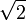$\sqrt{2}$ are both irrational. This set does not share any member with the rational number set.
5. The real numbers ($\mathbb{R}$) find applications in measurements and advanced mathematics. They are usually best written as decimal numbers (e.g., the value of e is approximately equal to 2.718281828). This set includes the rational numbers and the irrational numbers.
6. The complex numbers ($\mathbb{C}$) have two parts, where one is real and the other is some number multiplied by the imaginary number$i\!$, which is defined as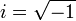$i = \sqrt{-1}$. The complex numbers were discovered while searching solutions to some polynomials (e.g., the polynomial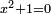$x^2 + 1 = 0$ has two solutions, one being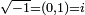$\sqrt{-1} = (0, 1) = i$). Because the complex number set is algebraically closed, it finds applications in many scientific fields, such as engineering and applied mathematics. This set includes the real numbers.
7. A complex number that is solution to a polynomial in integer coefficients is an algebraic number. This set includes all rational numbers and a subset of the irrational numbers. Any other complex number is a transcendental number.

In order to meet their needs, scientists created other number sets. To ease the study of quadratic forms, Carl Friedrich Gauss introduced from 1829 to 1831 what is known today as the gaussian integers. While studying 3D mechanics, William Rowan Hamilton introduced the quaternions in 1843 (today, they are largely superseded by vectors). Octonions were discovered in 1843. Georg Cantor, through its naive set theory, formally defined the notion of infinity in 1895. Kurt Hensel first described the p-adic numbers in 1897, looking for a way to bring the ideas and the techniques of power series within number theory.

We can consider unitless vectors and unitless matrices as number sets, since they mathematically abstract phenomenas in a unique way and we can apply operations upon them.

##  Notation

The notation plays a central role in the perception of what a number is and what we can do with it. A good notation saves lots of work when operating on numbers (and more generally on any mathematical abstract objects). For instance, it is possible to add numbers written in roman numerals (e.g., MCMXCVIII plus CCXVII). However, it is faster to add numbers written in base 10 (e.g., 1998 plus 217). The gain is higher when multiplying numbers.

In the Western world, the positional number system in base ten is the most used number notation. In this system, a numeral is constructed by putting digits side by side, each position in the numeral having a different numerical weight (a power of 10). In some knowledge fields, other numeral systems allow better handling of information. For instance, electronic engineers use binary numbers when dealing with electronic circuits.

• Integer numerals are prepended with the minus or the plus symbol ("-" and "+"). This applies to any numeral, as long as it does not represent a natural number.
• Numerals may come with a radix point, the decimal separator in base 10 (the period "." in some systems, the comma "," in others).
• In long numerals, digits are grouped and may contain a thousand separator (e.g., the speed of light in vacuum is written as 1,079,252,849 km/h in some systems, while it is written as 1 079 252 849 km/h in some others).
• Percentages ("%") allow to write a numeral as a fraction with the denominator 100 (e.g., 14.5% =$\frac{14.5}{100}$).
• Per mills ("‰") allow to write a numeral as a fraction with the denominator 1,000 (e.g., 22.3‰ =$\frac{22.3}{1000}$).
• Per cent mille (pcm) allow to write a numeral as a fraction with the denominator 100,000 (e.g., 78.7 pcm =$\frac{78.7}{100000}$).
• Parts per million (ppm), parts per billion (ppb) and parts per trillion (ppt) are others way to write a numeral as a fraction with the denominators 1 million, 1 billion, and 1 trillion.
• Very small and very large numbers are usually expressed in scientific notation. Their numeral uses the product symbol × or E (e.g., the speed of light in vacuum is approximately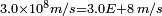$3.0 \times 10^8 m/s = 3.0 E+8 \, m/s$).

There are other ways to represent a number.

• Fractions contain a slash or a vinculum (e.g.,$a / b = \frac{a}{b}$). Ratios use the colon (e.g., 1.5 : 5).
• To shorten some numerals (or to show some properties), numbers are represented using an exponentation (e.g., 34), a radical symbol (e.g.,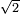$\sqrt 2$), a repeated pattern (e.g.,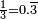$\frac{1}{3} = 0.\overline{3}$). Almost any expression having only functions and numerals may represent a number (e.g.,$\sin ( \frac{\pi}{3} )$,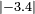$|-3.4|$, and$\zeta (3)$).
• Complex numbers are represented either by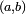$(a, b)$ or by$a + b i$, where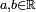$a, b \in \R$.
• In physics, vectors are usually represented as the sum of unit vectors :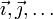$\vec{ \imath }, \vec{ \jmath }, \ldots$. In mathematics, we may encounter the "hat notation" :$\hat{ \imath }, \hat{ \jmath }, \ldots$
• Named constants are another way to represent numbers : π, e, γ, etc.

In geometry, a number can be represented in different ways. For instance, the length between two points in a cartesian coordinate system may represent a number. Fractions are sometimes represented by a rectangular grid. We could represent$\frac{7}{12}$ by a grid.

In statistics, numbers are represented by areas in histogram or by height in bar charts. In pie charts, values are proportional to the central angles. There are many other ways to represent numbers in statistics.

Many other scientific fields have their own notations.

There are cases where it is difficult to say if a symbol represents a number. Take for instance the units in International System of Units. When we write 30 cm, it means 30 ÷ 100 × meter. Officially, we should see "cm" as a centimeter, a unit of measure. However, 30 cm is the same as 0.3 m. For this reason, "30 c" represents a number: 0.3.Some content on this page may previously have appeared on Citizendium.